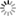##### Personal tools
You are here: Home AI and Calculus

# AI and Calculus

### - Overview

Calculus is a critical part of artificial intelligence (AI) and machine learning. Calculus is used to optimize algorithms, train models, and perform regularization. It also helps AI algorithms learn using the concept of gradient descent, which is based on the derivative from calculus.

Other branches of mathematics that are essential for AI include linear algebra, probability, and statistics. These topics are used together with computer programming to create AI.

AI algorithms and models use mathematics to process, analyze, and interpret large amounts of data.

### - Calculus and Integration

Calculus, originally called infinitesimal calculus or "infinitesimal calculus", is the mathematical study of continuous change, just as geometry is the study of shapes and algebra is the study of generalizations of arithmetic operations.

It has two main branches, calculus and integration; calculus focuses on the instantaneous rate of change and the slope of a curve, while integral calculus focuses on the accumulation of quantities, and the area under or between curves.

These two branches are related to each other through the Fundamental Theorem of Calculus, which exploits the fundamental concept of infinite sequences and infinite series converging to well-defined limits.

Infinitely small calculus was developed independently by Isaac Newton and Gottfried Wilhelm Leibniz in the late 17th century. Later work, including codifying the concept of limits, placed these developments on a more solid conceptual basis. Today, calculus has a wide range of uses in science, engineering, and the social sciences.

### - AI and Calculus

Calculus deals with changes in parameters, functions, errors, and approximations. A working knowledge of multidimensional calculus is essential in artificial intelligence.

Here are the most important concepts in calculus (though not exhaustive):

• Derivative  —  rules (addition, product, chain rule, etc.), hyperbolic derivatives (tanh, cosh, etc.), and partial derivatives.
• Vector/Matrix Calculus  —  Different Derivative Operators (Gradient, Jacobian, Hessian, and Laplace)
• Gradient Algorithms  —  local/global maxima and minima, saddle points, convex functions, batch and mini-batch, stochastic gradient descent, and performance comparisons.

### - Essential Topics in Calculus

Here is a list of essential topics in Calculus:

• Functions: a relationship between two variables, an independent variable
and a dependent variable.
• Scalar derivative: definition, intuition, common rules of differentiation, chain rule, partial derivatives.
• Gradient: concept, intuition, properties, directional derivative.
• Vector and matrix calculus: how to find derivative of {scalar-valued, vector-valued} function wrt a {scalar, vector} -> four combinations- Jacobian.
• Gradient algorithms: local/global maxima and minima, saddle point, convex functions, gradient descent algorithms- batch, mini-batch, stochastic, their performance comparison.

Please refer to the following for more details.

[More to come ...]

##### Document Actions